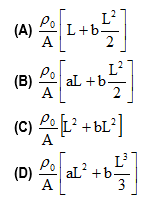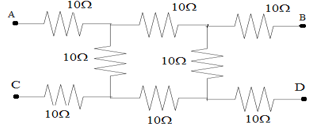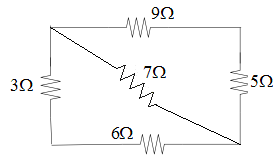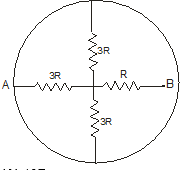Multiple Choice questions on Electric current resistance and resistivity for Jee Main and Advanced

Assertion and Reason Type Question
Following question consider two statements one labelled as statement-1 and another as statement-2. Study both statements and mark your answer using the codes given blow.
1. Both Statement-1 and Statement-2 are true and Statement-2 is correct explanation of Statement-1.
2.  Both Statement-1 and Statement-2 are true and Statement-2 is not a correct explanation of Statement-1.
3. Statement-1 is true but statement-2 is false.
4. Statement-1 is false and statement-2 is true.
Question-1
Statement-1 Free electrons in conductor are always in state of continuous random motion.
Statement-2 The random motion of free electrons is due to thermal energy of the conductor.
Question-2
Statement-1 Drift velocity of electrons in a conductor increase on increasing the temperature of the conductor.
Statement-2 On increasing the temperature of a conductor, resistivity of the conductor increases.
Question-3
Statement-1 For metals relaxation time for electrons decreases with increase in temperature.
Statement-2 With the increase in temperature, number of collision per unit time that electrons made with lattice ion also increases.

Multiple Choice Type Questions

Question-4
Resistivity of the material of a conductor having uniform area of cross-section varies along its length according to the relation
ρ= ρ0 (a+bx)
if L is the length of the conductor and be the area of cross-section then resistivity of the conductor given by relationQuestion-5
Given a current carrying wire of non-uniform cross-section. Which of the following is constant through out the wire.
(A) Current only
(B) Current and drift speed
(C) Drift speed only
(D) Current, drift speed and electric field

Question-6
When potential difference across a given copper wire is increase, drift velocity of charge carriers
(A) Decreases
(B) Increases
(C) Remain same
(D) Get reduced to zero

Question-7
A material B has twice the specific resistance of the material A. A circular wire made of B has twice the diameter of the wire made of A. Then , for the two wires to have to have the same resistance, the ratio
La/Lb of their respective lengths must be
(A) ½
(B) 2
(C) ¼
(D) 1

Question-8
What will be the equivalent resistance between A and D(A) 40  Ω
(B) 20Ω
(C) 30   Ω
(D) 10Ω

Question-9
In the given circuit below the equivalent resistance between points A and C(A) 32
(B) 30.7
(C) 33.07
(D) 3.07

Question-10
A circular ring having negligible resistance is used to connect four resistors as shown below in the figure.
The equivalent resistance between point A and B is(A) 10R
(B) 2R
(C) 3R
(D) R